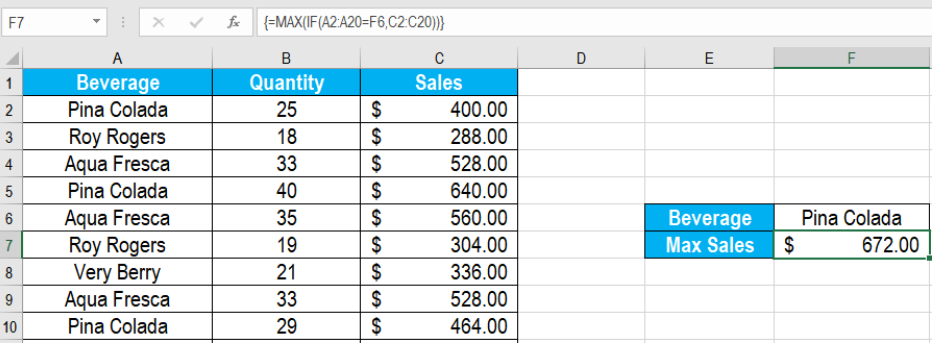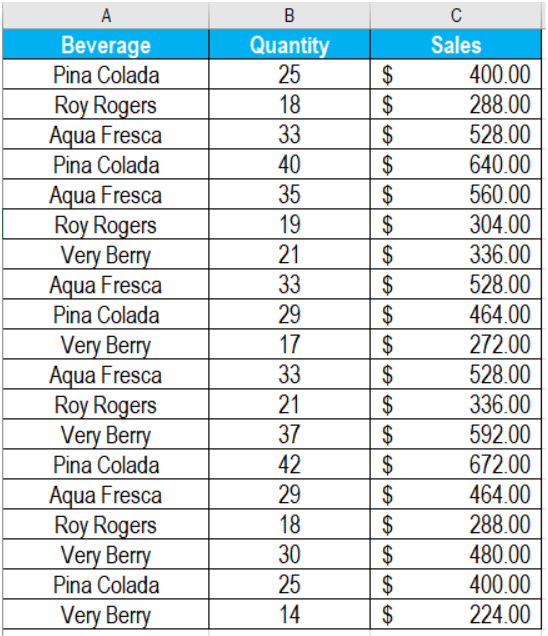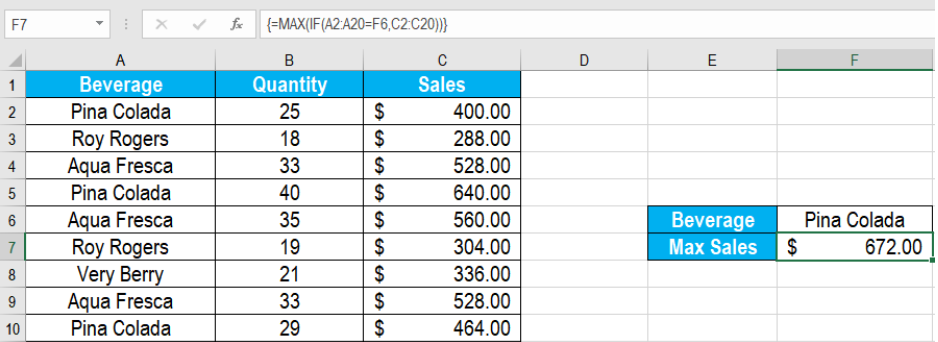Get instant live expert help with Excel or Google Sheets“My Excelchat expert helped me in less than 20 minutes, saving me what would have been 5 hours of work!”

#### Post your problem and you’ll get Expert help in seconds.

Your message must be at least 40 characters
Our professional Expert are available now. Your privacy is guaranteed.

# How to use the Max if Criteria Match

We often work with ranges in Excel where we need to find the largest value based on certain criteria. The MAX IF criteria match helps us to find the largest value within a range of cells based on a specific condition. In this tutorial, we will learn how to use the MAXIF criteria match in Excel.Figure 1. Example of Using the Max if Criteria Match

## Generic Formula

`{=MAX(IF(criteria_range=criteria,value_range))}`

• Criteria_range: This is a required value. It is an array of heterogeneous values contain subsets of homogeneous values. It is the filter boundary.
• Criteria: This value is required as well. It is the actual value that we check in the criteria range.
• Value_range: Value_range is also a required value. This is the array list of values. We look to find the largest from this array based on the criteria from the criteria_range.

## How this formula works

This formula is based on the MAX and IF functions. First, IF tests whether the criteria_range contains the criteria value. As we compare the criteria with an array, Excel will return an array as the result. This array will contain the logical values TRUE or FALSE. The TRUE values will exist where the criteria exists within the criteria_range. The IF function will return an array consisting of the values from value_range. Here the criteria exists in the criteria_range. Finally, MAX extracts the max value from this array returned by IF.

## Setting Up Data

The following example uses a beverage sale data set. Column A, B and C has the beverage names, quantity and sales.Figure 2. The Sample Beverage Sales Data Set

To find the largest revenue among the beverages in column C:

• Go to cell F7.
• Assign the formula `=MAX(IF(A2:A20=F6,C2:C20))` to cell F5.
• Press CTRL + SHIFT + ENTER as it is an array formula.Figure 3. Applying the Formula to the data

This will show the max sales among the beverages in F7.

Most of the time, the problem you will need to solve will be more complex than a simple application of a formula or function. If you want to save hours of research and frustration, try our live Excelchat service! Our Excel Experts are available 24/7 to answer any Excel question you may have. We guarantee a connection within 30 seconds and a customized solution within 20 minutes.

### Did this post not answer your question? Get a solution from connecting with the expert.Another blog reader asked this question today on Excelchat:
Solution examplesCould you help me by explaining the IF function?
Solved by G. L. in 24 minsI have a question about Conditional Formatting. =IF('Sheet1'!A1="X",IF('Sheet1'!B1="Y", TRUE, FALSE),FALSE) the set the cell background to red. I would like to make it so that if Sheet1'!B1="Z" I set the back to blue. How can I achieve this.
Solved by B. F. in 39 minsI need to find out if the 2018 yearly sales goals were met if the yearly sales were \$25,000 or more using an IF logical function and to set the formula to return a value of YES if met, and NO if not
Solved by A. A. in 18 minsI filtered data in column A (it is labeled ID #) of a data set. On my next worksheet, column A is also the ID #, but it is a different data set. I want to filter the ID #'s the same for the two sheets, but how do I do that being that I am working with two different sets of data?
Solved by V. H. in 22 minsi want to create a very simple if function in a cell which is, if one cell is less than 1250, then the automatic value in the current cell is 250, dead easy, for someone who knows! Thank you.
Solved by A. H. in 14 mins## Subscribe to Excelchat.coAnother blog reader asked this question today on Excelchat: